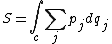least-action principle

Also found in: Dictionary.

Least-action principle

Like Hamilton's principle, the principle of least action is a variational statement that forms a basis from which the equations of motion of a classical dynamical system may be deduced. Consider a mechanical system described by coordinates q1, . . . , qf and their canonically conjugate momenta p1, …, pf. The action S associated with a segment of the trajectory of the system is defined by the equation below,where the integral is evaluated along the given segment c of the trajectory. The action is of interest only when the total energy E is conserved. The principle of least action states that the trajectory of the system is that path which makes the value of S stationary relative to nearby paths between the same configurations and for which the energy has the same constant value. The principle is misnamed, as only the stationary property is required. It is a minimum principle for sufficiently short but finite segments of the trajectory. See Hamilton's equations of motion, Hamilton's principle, Minimal principles

least-action principle

[¦lēst ′ak·shən ‚prin·sə·pəl]
(mechanics)
Site: Follow: Share:
Open / Close